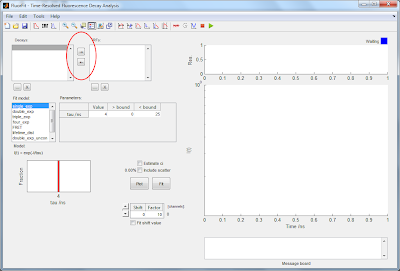### Format input data in version 1.2 and earlier

#### DecayFit version 1.0-1.2 accepts ASCII-formatted data files with the following data structures:

1) A single column data structure will be interpreted as an intensity vector (decay or IRF depending on which is being loaded). The program will ask the user to specify the channel time width when loading the data.

2) A two column data structure will be interpreted as:
Column 1: Time (ns)
Column 2: Intensity (decay or IRF depending on which is being loaded)

3) A three column data structure will be interpreted as:
Column 1: Time (ns)
Column 2: Decay
Column 3: IRF
This definition is applied independent of whether an IRF or a decay is being loaded.

4) A four column data structure will be interpreted as:
Column 1: Time decay (ns)
Column 2: Decay
Column 3: Time IRF (ns)
Column 4: IRF
This definition is applied independent of whether an IRF or a decay is being loaded.

- If the file contains more than four columns, column data above four will not be read and the first four columns will be interpreted according to case 4) above.

- In all cases, texted headerlines are allowed in the data files.

- Data definitions can also be changed after loading the data using the '<-' and '->' buttons (interchanging IRF and decay):